# Electricity or Gasoline? Visualizing vehicle lifetime fuel costs

[vc_row][vc_column][kleo_gap size=”60px”][vc_column_text]

# Electricity or gasoline? Visualizing vehicle lifetime fuel costs.

By Jed Bailey | October 18, 2018

Energy Narrative has developed a set of graphics to help visualize the money spent fueling an electric vehicle (EV) compared to a vehicle with an internal combustion engine (noted as an ICE in this report). Fuel cost is the single most important differentiator in the operational costs of EVs and ICEs. Fixed operating costs—insurance, title, taxes and the like—and other variable operating costs—primarily tires and maintenance—are generally similar for both technologies.

Therefore, understanding the difference in lifetime fuel cost is important when comparing the overall cost of vehicle ownership for EV’s and ICE’s. Since EV’s currently tend to have a higher purchase price than similar ICE’s, the difference in lifetime fuel expenses can indicate the maximum price premium that one would be willing to pay for an EV.

Our graphical approach to determining the lifetime fuel expense of a particular vehicle splits the calculation into two steps: first, determining the vehicle’s cost per mile, then applying this cost per mile to determine the lifetime fuel expense. This report describes the methodology and assumptions behind this approach.

Each step is illustrated with two theoretical examples: an EV with an average fuel efficiency of 4 miles per kWh and an average electricity price of US\$0.16 per kWh and an ICE with an average fuel efficiency of 30 miles per gallon and an average fuel price of US\$3.00 per gallon. Both vehicles are assumed to drive 20,000 miles per year and have a 10 year useful life.

The first step is to calculate the vehicle’s fuel cost per mile. In equation form, this is the average fuel price (electricity, gasoline, or diesel) times the vehicle’s fuel efficiency. Owing to the large difference in unit fuel costs for electricity and gasoline, we have split this step into two graphics: one that applies to ICEs and one that applies to EVs.

Figure 1 below calculates the average cost per mile for an ICE based on the expected average fuel price (US\$ per gallon) and the vehicle’s average fuel efficiency (miles per gallon) in graphical format. First, the user finds the point at which the average fuel price (Y coordinate) and the vehicle’s fuel efficiency (X coordinate) intersect. The intersection point is then compared to the diagonal cost per mile indicators to estimate the resulting value.

#### Figure 1: ICE average cost per mile calculation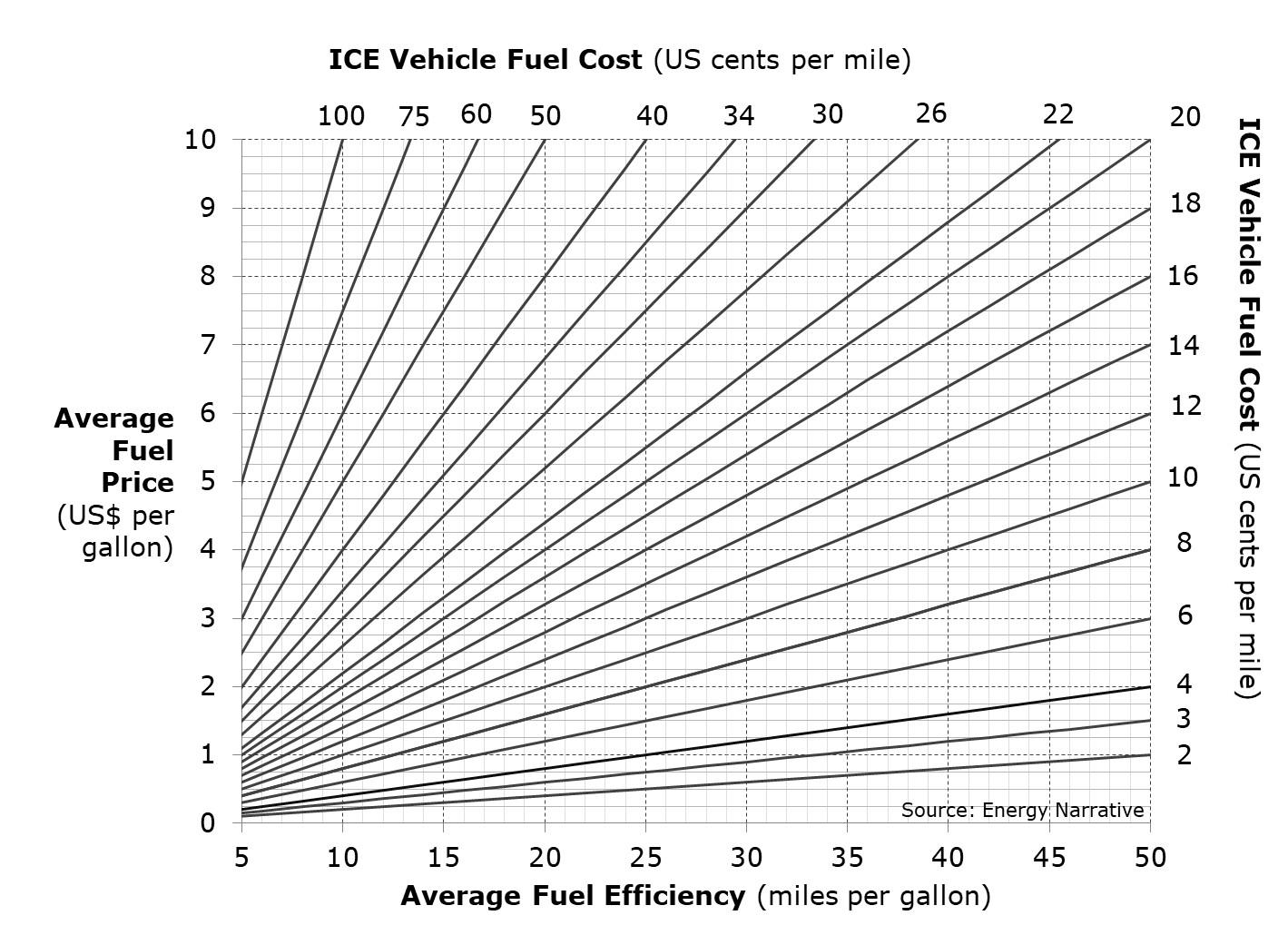Figure 2 below shows how to use the graphic with our example ICE vehicle (in red, of course). In this case, the 30 mpg average fuel efficiency intersects with the US\$3.00 per gallon average fuel price at the 10 cents per mile diagonal line.

#### Figure 2: Example ICE average cost per mile calculation, in red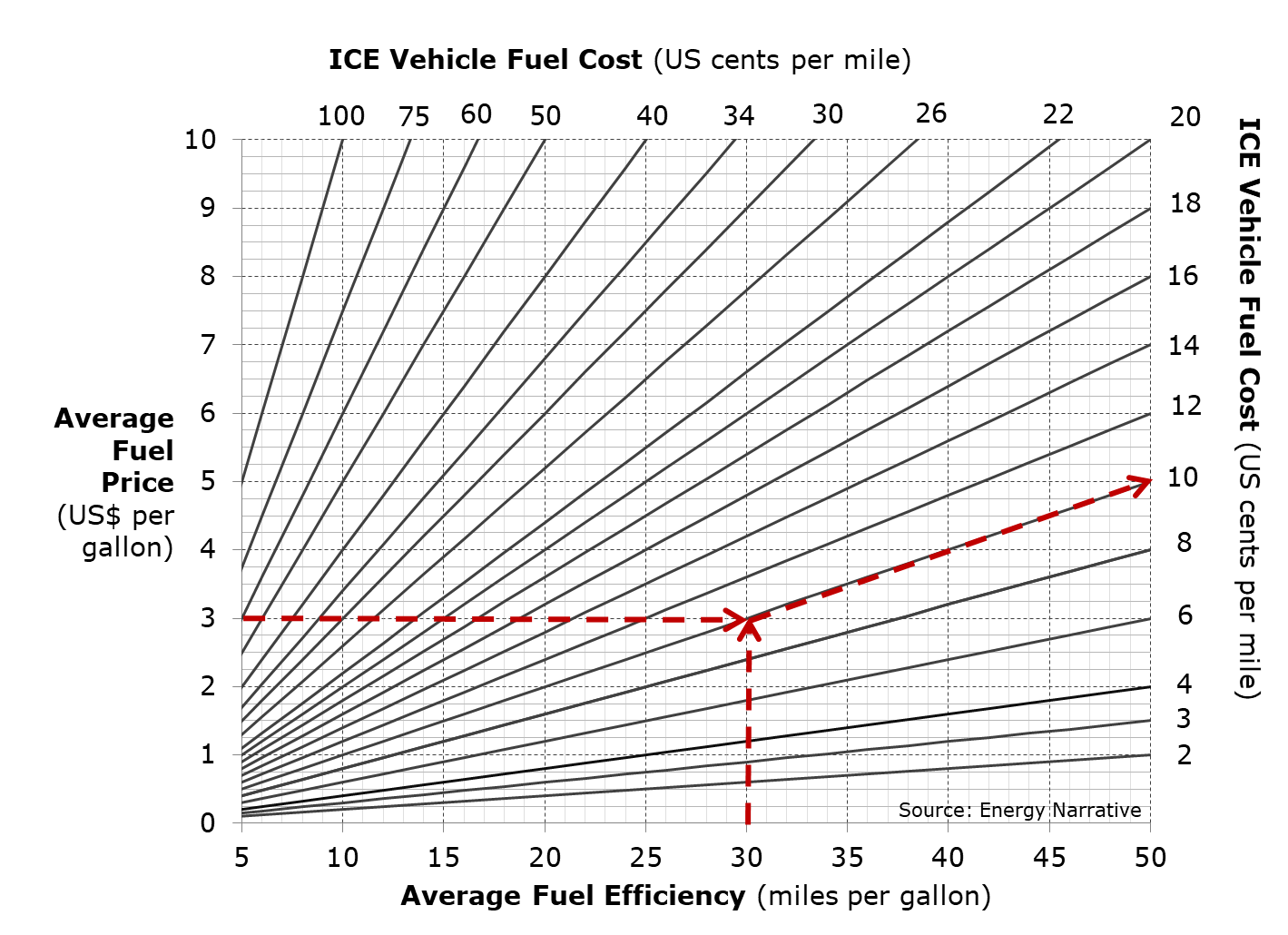Figure 3 below is a graphical representation of the same cost per mile calculation, performed for an EV. In this case, the average electricity price per kWh replaces the fuel price and the EV’s fuel efficiency is denominated in miles per kWh of electricity consumed.

#### Figure 3: EV average cost per mile calculation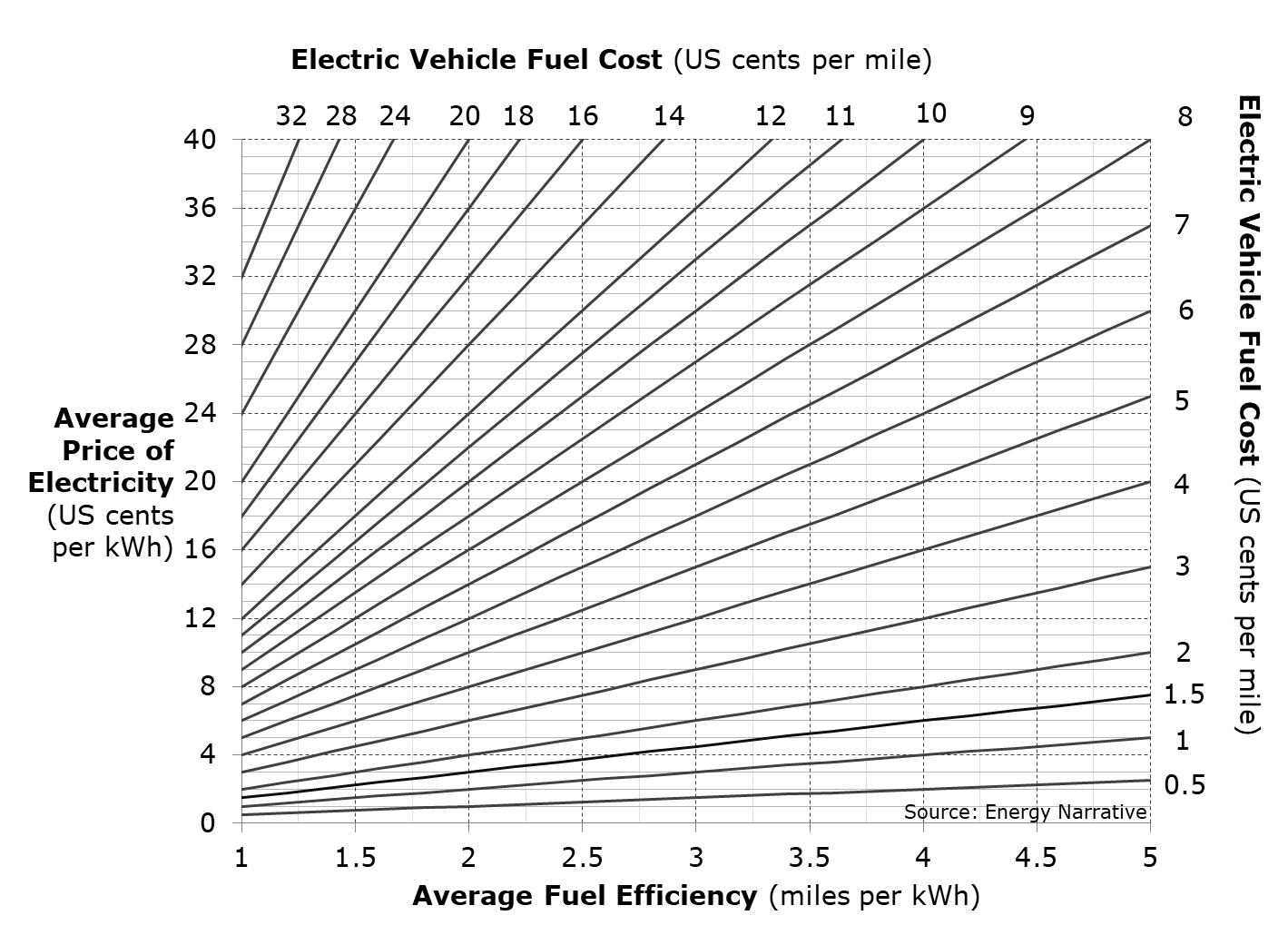Figure 4 below shows how to use the graphic with our example EV vehicle (in green, naturally). In our theoretical example, the EV travels 4 miles per kWh using electricity that cost 16 cents per kWh. These X and Y intercepts come together at the 4 cents per mile line.

#### Figure 4: Example EV average cost per mile calculation, in green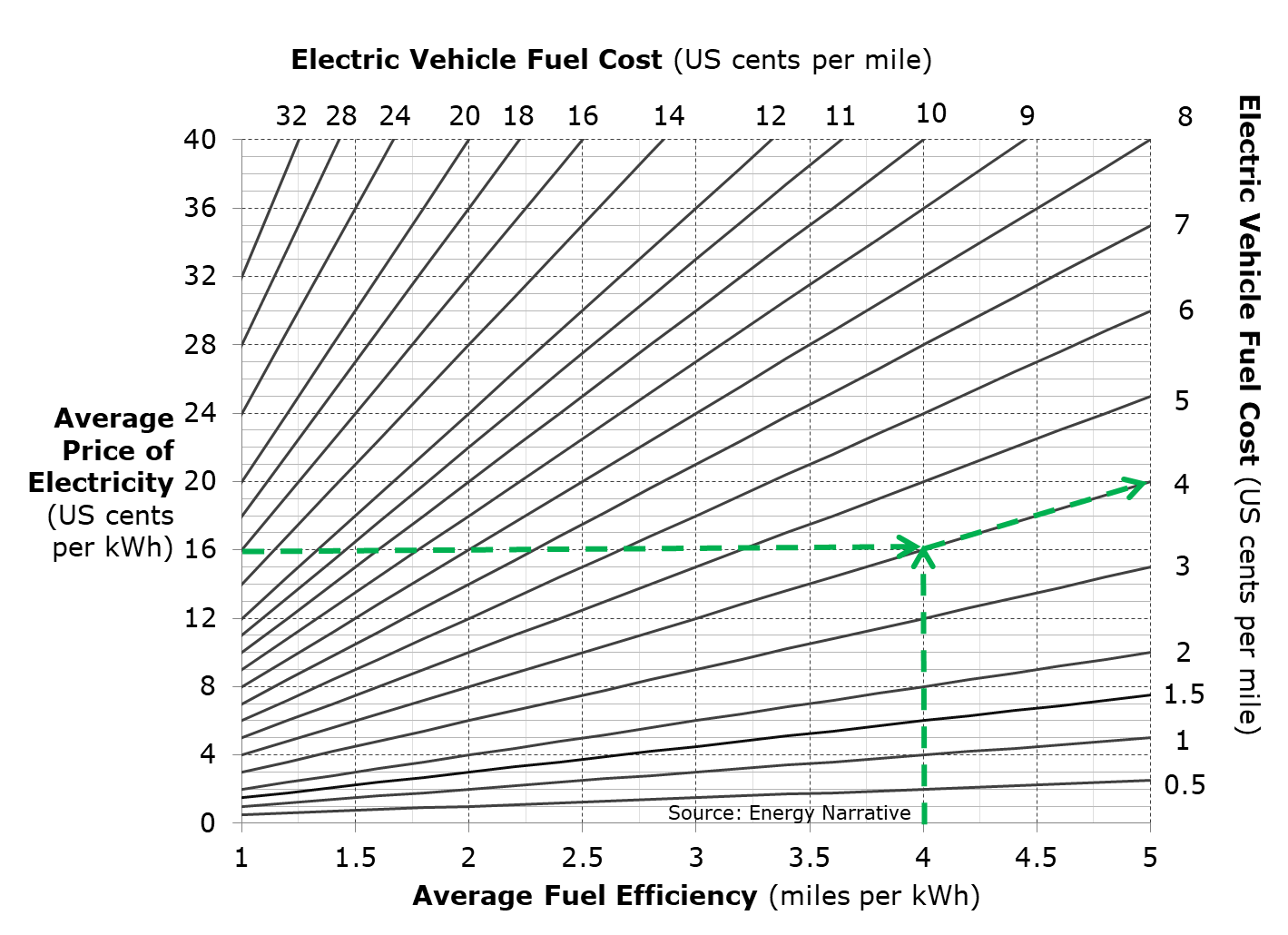Once the average cost per mile is established for any vehicle, one can calculate the total fuel cost over the vehicle’s 10-year life. In equation form, the total vehicle lifetime fuel cost is calculated as the fuel cost per mile times the number of miles traveled per year times the number of years the vehicle is driven.

Figure 5 below calculates the total fuel cost over a 10-year vehicle life in graphical form. First, locate the previously calculated vehicle cost per mile along the X-axis. Next, move vertically to intersect with the line representing the average number of miles that the vehicle travels each year. At this intersection, then move horizontally left to locate the Y-axis intercept representing the vehicle’s total fuel cost over 10 years.

Note that this value is the total amount of money spent over 10 years, expressed in real dollars. The calculation behind the graphic assumes that the fuel price is constant and does not make any adjustment for inflation. The X and Y axes are shown in log scale to better capture the range of costs.

#### Figure 5: Total vehicle fuel spend over 10 years (real dollars)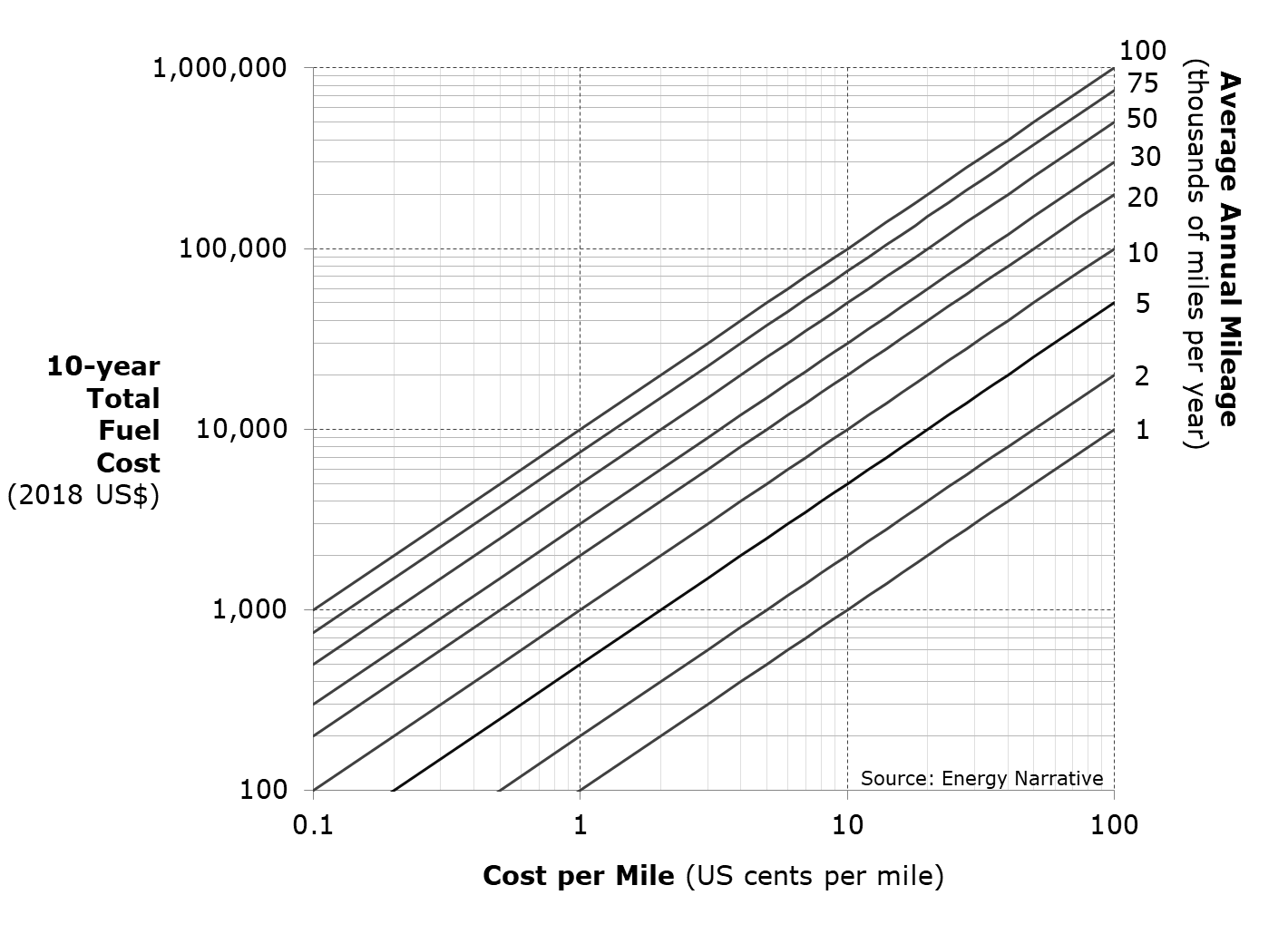Using the previous hypothetical examples, the owner of the 10-cent-per-mile ICE would spend \$20,000 on gasoline if they drove the car 20,000 miles per year for 10 years. The owner of the 4-cent-per-mile EV would spend US\$8,000 on electricity to drive the same distance over the same period. Both calculations are shown in Figure 6 below.

#### Figure 6: EV and ICE example total vehicle fuel spend over 10 years (real dollars)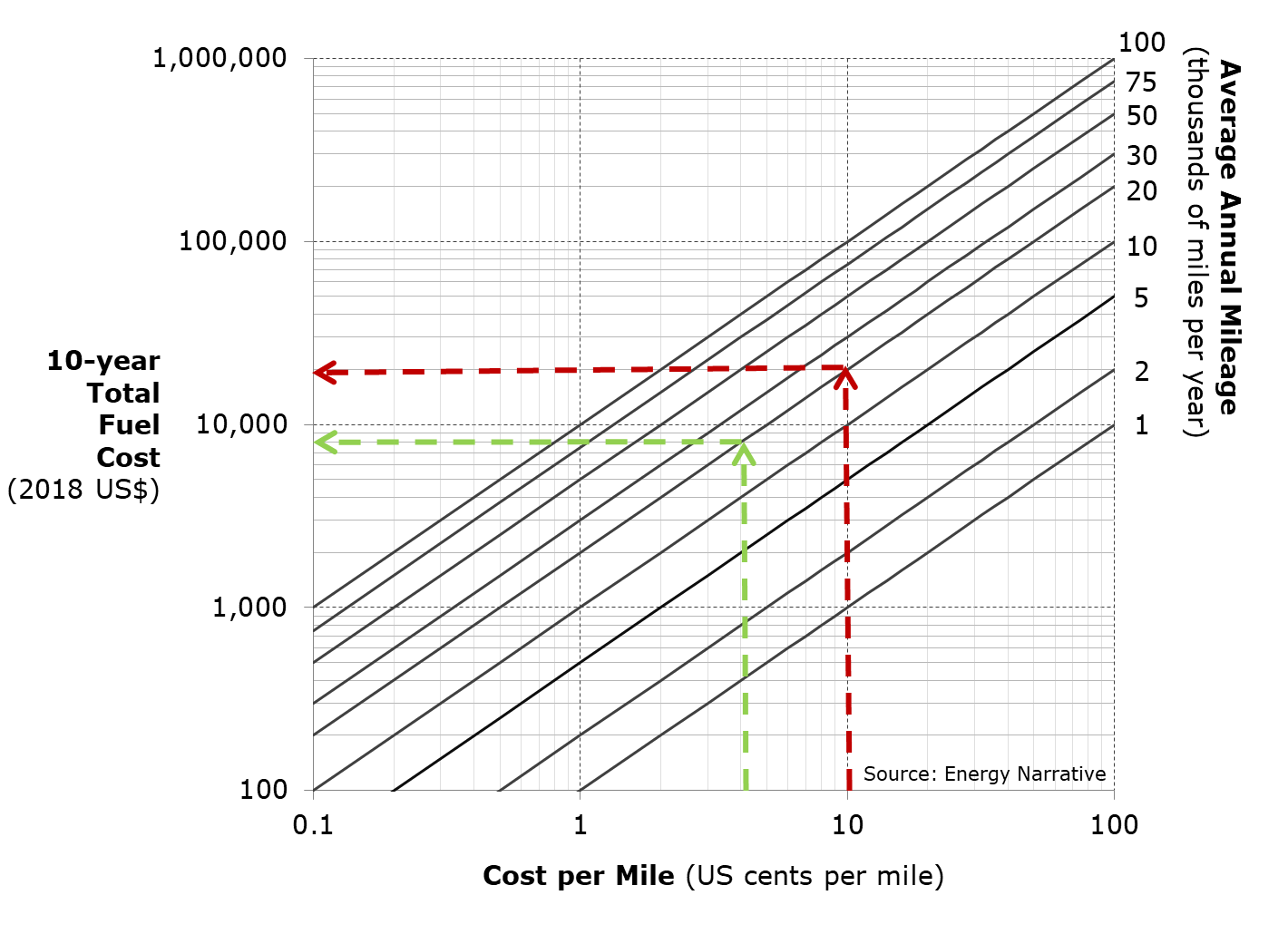Figure 5 above is useful to highlight the differences in the total amount of money spent to fuel EVs and ICEs over a 10-year life. In order to compare the relative overall cost to own each type of vehicle, however, the future fuel costs must be discounted to the present. This allows the stream of future fuel costs to be directly combined with the initial purchase price, resulting in the total present cost of owning each vehicle.

Figure 7 below performs the same calculation as Figure 5 above, but presents the results in net present value terms using a 10% real discount rate. This figure can be used to compare the net present value of the fuel costs for different vehicles. The resulting difference represents the present value of the savings that would accrue over the vehicle’s life to the owner of the vehicle with lower fuel costs relative to the vehicle with higher fuel costs. If other owner costs, such as insurance and maintenance, are the same for both vehicles, the difference in fuel costs represents the maximum premium in initial purchase price that one would be willing to pay for the vehicle with lower fuel costs.

#### Figure 7: Net present value of vehicle fuel costs over 10 years (10% real discount rate)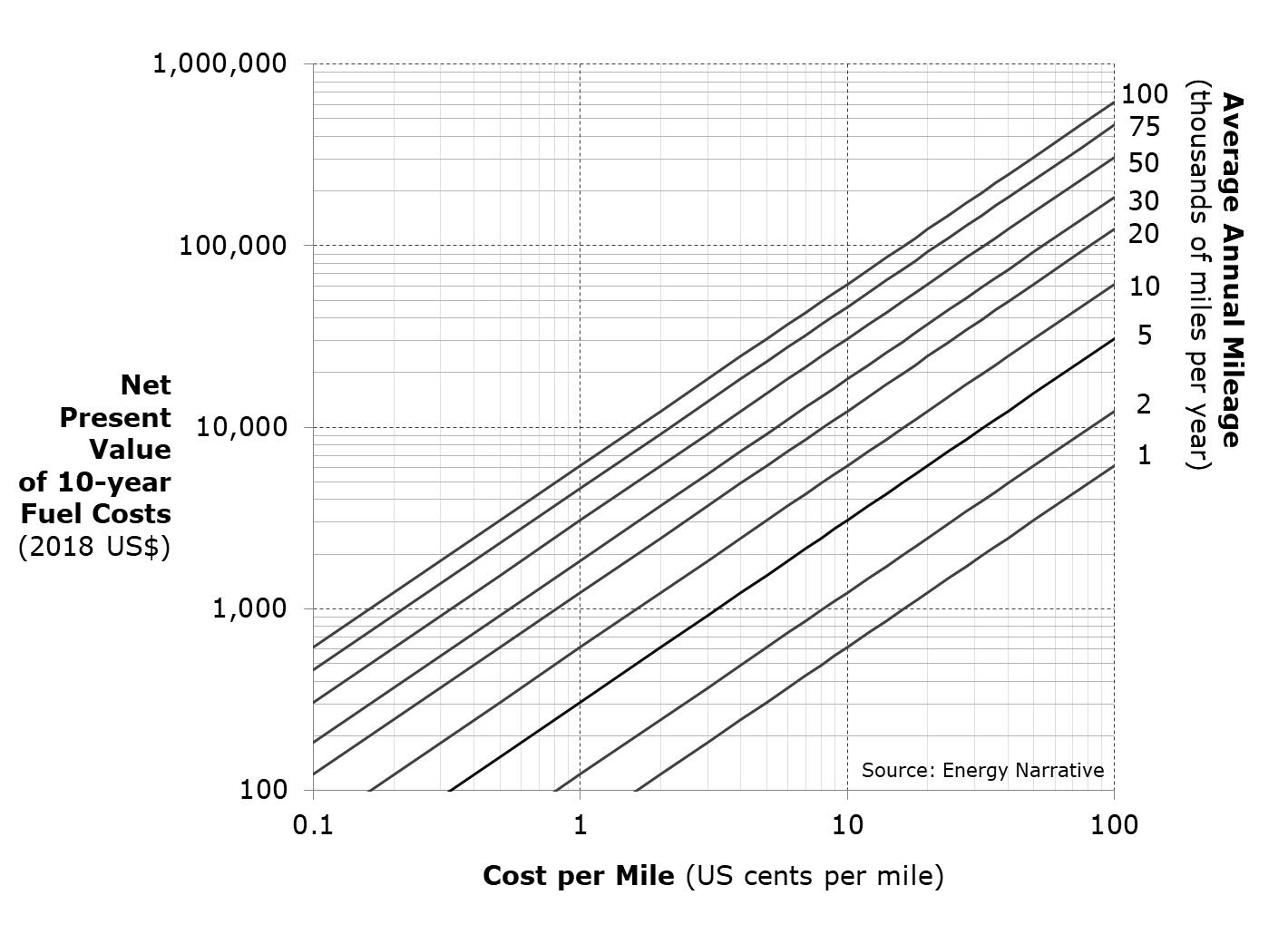In terms of our hypothetical EV and ICE examples, Figure 8 below shows that the present value of the gasoline purchased over 10 years for the 10-cent-per-mile ICE is just over US\$12,000. The present value of the electricity purchased for the 4-cent-per-mile EV over the same period is almost US\$5,000. This suggests that a buyer should be willing to pay up to US\$7,000 more for the hypothetical EV than for the hypothetical ICE (assuming other costs are the same for both).

#### Figure 8: EV and ICE example net present value of vehicle fuel costs over 10 years (10% real discount rate)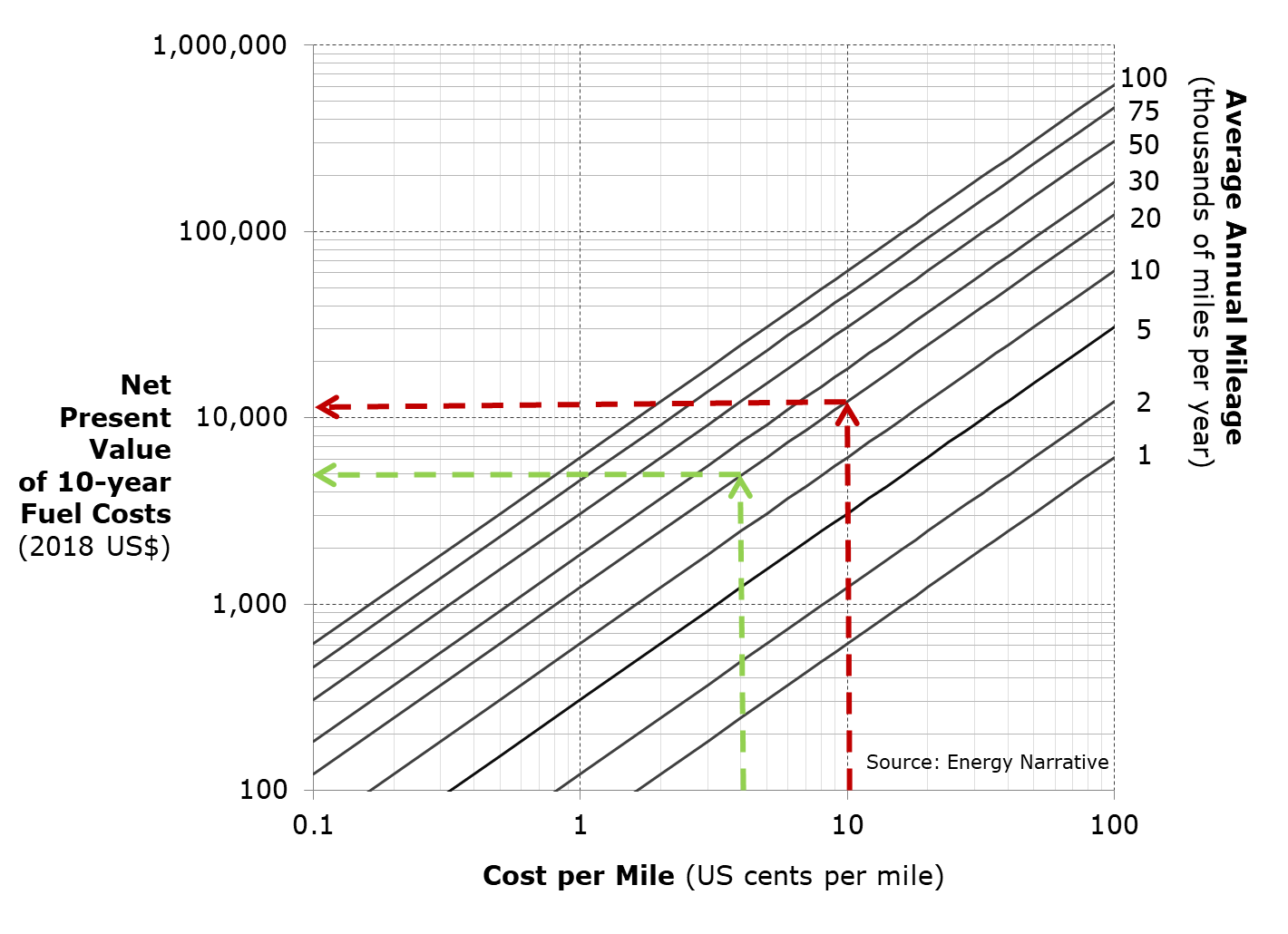As noted above, fuel is not the only cost for vehicle owners. If other costs are significantly different, the relative economics of owning each vehicle would be skewed from what is shown in the graphic. This analysis is also fixed at a 10% real discount rate (roughly 12% nominal discount rate at today’s inflation rate). This discount rate many not be applicable to in all cases. Another limitation of the approach is the assumption that fuel prices are constant (in real terms) over time. In reality, fuel prices are volatile, and liquid fuel prices tend to be more volatile than electricity prices. The analysis does not capture the value of this reduced volatility.

Nevertheless, the approach provides a simple way to visualize how differences in vehicle efficiency, fuel prices, and vehicle usage affect overall owner costs over a range of technologies and fuels. Energy Narrative will use these charts to explore the relative cost of EVs and ICEs under different circumstances in a series of upcoming reports. A list of related reports will be added below and updated with a link to each new report as they are completed.